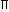# Aptitude - Volume and Surface Area

Exercise : Volume and Surface Area - General Questions
6.
A boat having a length 3 m and breadth 2 m is floating on a lake. The boat sinks by 1 cm when a man gets on it. The mass of the man is:
12 kg
60 kg
72 kg
96 kg
Explanation:

 Volume of water displaced = (3 x 2 x 0.01) m3 = 0.06 m3.Mass of man = Volume of water displaced x Density of water = (0.06 x 1000) kg = 60 kg.

7.
50 men took a dip in a water tank 40 m long and 20 m broad on a religious day. If the average displacement of water by a man is 4 m3, then the rise in the water level in the tank will be:
20 cm
25 cm
35 cm
50 cm
Explanation:

Total volume of water displaced = (4 x 50) m3 = 200 m3.Rise in water level =200m 0.25 m = 25 cm. 40 x 20

8.
The slant height of a right circular cone is 10 m and its height is 8 m. Find the area of its curved surface.
30m2
40m2
60m2
80m2
Explanation:

l = 10 m,

h = 8 m.

So, r = l2 - h2 = (10)2 - 82 = 6 m.Curved surface area =rl = (x 6 x 10) m2 = 60m2.

9.
A cistern 6m long and 4 m wide contains water up to a depth of 1 m 25 cm. The total area of the wet surface is:
49 m2
50 m2
53.5 m2
55 m2
Explanation:
 Area of the wet surface = [2(lb + bh + lh) - lb] = 2(bh + lh) + lb = [2 (4 x 1.25 + 6 x 1.25) + 6 x 4] m2 = 49 m2.

10.
A metallic sheet is of rectangular shape with dimensions 48 m x 36 m. From each of its corners, a square is cut off so as to make an open box. If the length of the square is 8 m, the volume of the box (in m3) is:
4830
5120
6420
8960Volume of the box = (32 x 20 x 8) m3 = 5120 m3.